# 410. 分割数组的最大值# 301. 删除无效的括号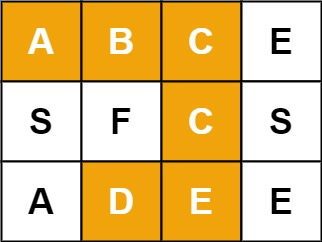# 79. 单词搜索

Given an m x n grid of characters board and a string word, return true if word exists in the grid.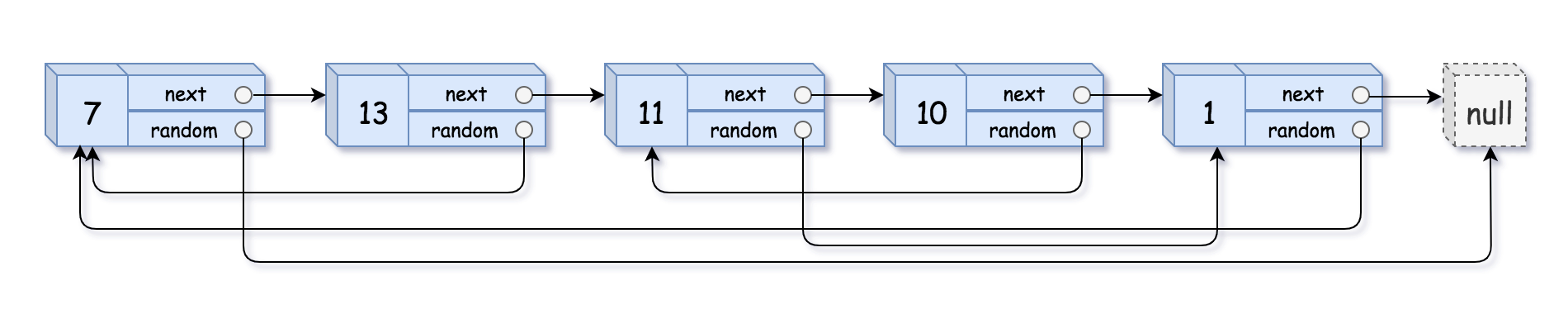# 138. Copy List with Random Pointer

A linked list of length n is given such that each node contains an additional random pointer, which could point to any node in the list, or null.Construct a deep copy of the list.# 1143. Longest Common Subsequence

Given two strings text1 and text2, return common subsequence. If there is no common subsequence, return 0.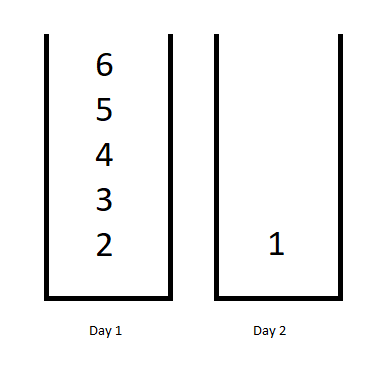# 1335. Minimum Difficulty of a Job Schedule

You want to schedule a list of jobs in d days. Jobs are dependent (i.e To work on the i-th job, you have to finish all the jobs j where 0 <= j < i).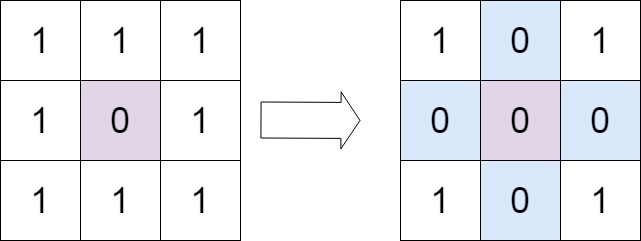# 73. Set Matrix Zeroes

Given an m x n matrix. If an element is 0, set its entire row and column to 0. Do it in-place.# 重排奇偶有序链表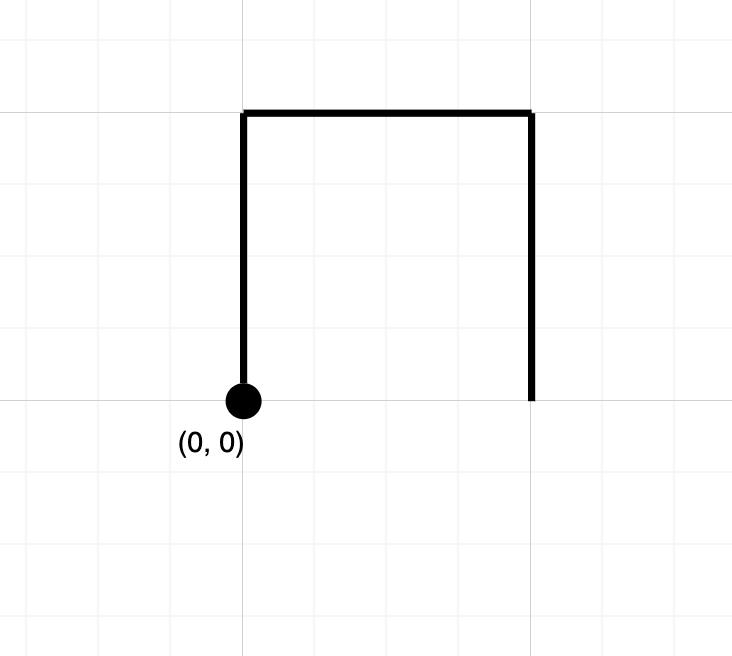# 1496. Path Crossing

Return True if the path crosses itself at any point, that is, if at any time you are on a location you've previously visited. Return False otherwise.# 680. Valid Palindrome II

Given a non-empty string s, you may delete at most one character. Judge whether you can make it a palindrome.# String - 71. Simplify Path

Given an absolute path for a file (Unix-style), simplify it. Or in other words, convert it to the canonical path.# String - 67. Add Binary

Given two binary strings, return their sum (also a binary string).# Math - 50. Pow(x, n)

Implement pow(x, n), which calculates x raised to the power n (xn).# String - 44. Wildcard Matching

Given an input string (s) and a pattern (p), implement wildcard pattern matching with support for '?' and '*'.# DFS&BFS - 37. Sudoku Solver

Write a program to solve a Sudoku puzzle by filling the empty cells.# Array - 36. Valid Sudoku

Determine if a 9x9 Sudoku board is valid.# Array - 57. Insert Interval

Given a set of non-overlapping intervals, insert a new interval into the intervals (merge if necessary).# Binary Search - 378. Kth Smallest Element in a Sorted Matrix

Given a n x n matrix where each of the rows and columns are sorted in ascending order, find the kth smallest element in the matrix.# Array - 59. Spiral Matrix II

Given a positive integer n, generate a square matrix filled with elements from 1 to n2 in spiral order.# Array - 54. Spiral Matrix

Given a matrix of m x n elements (m rows, n columns), return all elements of the matrix in spiral order.

Nothing just happens, it's all part of a plan.